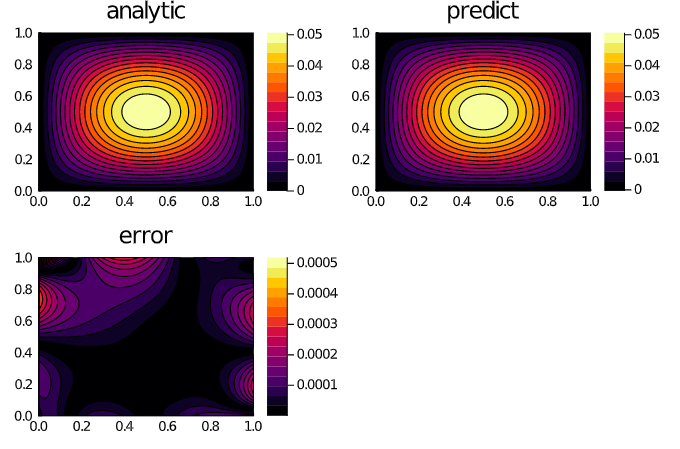# Poisson Equation

In this example, we will solve a Poisson equation:

$$$∂^2_x u(x, y) + ∂^2_y u(x, y) = - \sin(\pi x) \sin(\pi y) \, ,$$$

with the boundary conditions:

\begin{align*} u(0, y) &= 0 \, ,\\ u(1, y) &= - \sin(\pi) \sin(\pi y) \, ,\\ u(x, 0) &= 0 \, ,\\ u(x, 1) &= - \sin(\pi x) \sin(\pi) \, , \end{align*}

on the space domain:

$$$x \in [0, 1] \, , \ y \in [0, 1] \, ,$$$

with grid discretization dx = 0.1. We will use physics-informed neural networks.

## Copy-Pastable Code

using NeuralPDE, Flux, ModelingToolkit, Optimization, GalacticOptimJL, DiffEqFlux
import ModelingToolkit: Interval, infimum, supremum

@parameters x y
@variables u(..)
Dxx = Differential(x)^2
Dyy = Differential(y)^2

# 2D PDE
eq  = Dxx(u(x,y)) + Dyy(u(x,y)) ~ -sin(pi*x)*sin(pi*y)

# Boundary conditions
bcs = [u(0,y) ~ 0.0, u(1,y) ~ -sin(pi*1)*sin(pi*y),
u(x,0) ~ 0.0, u(x,1) ~ -sin(pi*x)*sin(pi*1)]
# Space and time domains
domains = [x ∈ Interval(0.0,1.0),
y ∈ Interval(0.0,1.0)]

# Neural network
dim = 2 # number of dimensions
chain = FastChain(FastDense(dim,16,Flux.σ),FastDense(16,16,Flux.σ),FastDense(16,1))
# Initial parameters of Neural network
initθ = Float64.(DiffEqFlux.initial_params(chain))

# Discretization
dx = 0.05
discretization = PhysicsInformedNN(chain,GridTraining(dx),init_params =initθ)

@named pde_system = PDESystem(eq,bcs,domains,[x,y],[u(x, y)])
prob = discretize(pde_system,discretization)

#Optimizer
opt = GalacticOptimJL.BFGS()

#Callback function
callback = function (p,l)
println("Current loss is: $l") return false end res = Optimization.solve(prob, opt, callback = callback, maxiters=1000) phi = discretization.phi using Plots xs,ys = [infimum(d.domain):dx/10:supremum(d.domain) for d in domains] analytic_sol_func(x,y) = (sin(pi*x)*sin(pi*y))/(2pi^2) u_predict = reshape([first(phi([x,y],res.minimizer)) for x in xs for y in ys],(length(xs),length(ys))) u_real = reshape([analytic_sol_func(x,y) for x in xs for y in ys], (length(xs),length(ys))) diff_u = abs.(u_predict .- u_real) p1 = plot(xs, ys, u_real, linetype=:contourf,title = "analytic"); p2 = plot(xs, ys, u_predict, linetype=:contourf,title = "predict"); p3 = plot(xs, ys, diff_u,linetype=:contourf,title = "error"); plot(p1,p2,p3) ## Detailed Description The ModelingToolkit PDE interface for this example looks like this: using NeuralPDE, Flux, ModelingToolkit, Optimization, GalacticOptimJL, DiffEqFlux import ModelingToolkit: Interval, infimum, supremum @parameters x y @variables u(..) @derivatives Dxx''~x @derivatives Dyy''~y # 2D PDE eq = Dxx(u(x,y)) + Dyy(u(x,y)) ~ -sin(pi*x)*sin(pi*y) # Boundary conditions bcs = [u(0,y) ~ 0.0, u(1,y) ~ -sin(pi*1)*sin(pi*y), u(x,0) ~ 0.0, u(x,1) ~ -sin(pi*x)*sin(pi*1)] # Space and time domains domains = [x ∈ Interval(0.0,1.0), y ∈ Interval(0.0,1.0)] Here, we define the neural network, where the input of NN equals the number of dimensions and output equals the number of equations in the system. # Neural network dim = 2 # number of dimensions chain = FastChain(FastDense(dim,16,Flux.σ),FastDense(16,16,Flux.σ),FastDense(16,1)) Convert weights of neural network from Float32 to Float64 in order to all inner calculation will be with Float64. # Initial parameters of Neural network initθ = Float64.(DiffEqFlux.initial_params(chain)) Here, we build PhysicsInformedNN algorithm where dx is the step of discretization, strategy stores information for choosing a training strategy and init_params =initθ initial parameters of neural network. # Discretization dx = 0.05 discretization = PhysicsInformedNN(chain, GridTraining(dx),init_params =initθ) As described in the API docs, we now need to define the PDESystem and create PINNs problem using the discretize method. @named pde_system = PDESystem(eq,bcs,domains,[x,y],[u(x, y)]) prob = discretize(pde_system,discretization) Here, we define the callback function and the optimizer. And now we can solve the PDE using PINNs (with the number of epochs maxiters=1000). #Optimizer opt = GalacticOptimJL.BFGS() callback = function (p,l) println("Current loss is:$l")
return false
end

res = Optimization.solve(prob, opt, callback = callback, maxiters=1000)
phi = discretization.phi

We can plot the predicted solution of the PDE and compare it with the analytical solution in order to plot the relative error.

xs,ys = [infimum(d.domain):dx/10:supremum(d.domain) for d in domains]
analytic_sol_func(x,y) = (sin(pi*x)*sin(pi*y))/(2pi^2)

u_predict = reshape([first(phi([x,y],res.minimizer)) for x in xs for y in ys],(length(xs),length(ys)))
u_real = reshape([analytic_sol_func(x,y) for x in xs for y in ys], (length(xs),length(ys)))
diff_u = abs.(u_predict .- u_real)

p1 = plot(xs, ys, u_real, linetype=:contourf,title = "analytic");
p2 = plot(xs, ys, u_predict, linetype=:contourf,title = "predict");
p3 = plot(xs, ys, diff_u,linetype=:contourf,title = "error");
plot(p1,p2,p3)